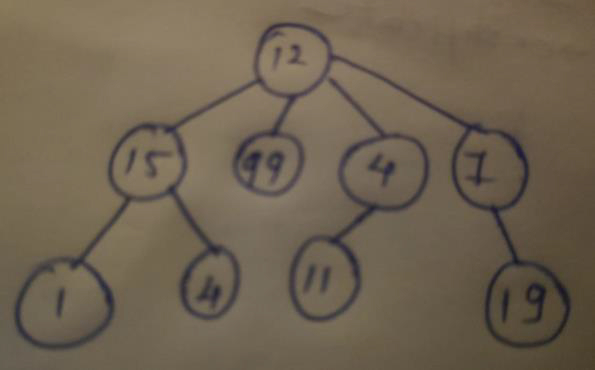# Preorder Traversal of N-ary Tree Without Recursion in C++

In this problem, we are given an N-ary Tree. Our task is to print the preorder traversal of the tree.

First, let’s learn some basic terminologies,

N-ary Tree is a tree in which all nodes can have at max N child nodes. Example 2-ary (binary) tree has max 2 child nodes.

Preorder Traversal is a way to traverse nodes of the tree. In this we will first traverse root node then left child and then the right child.

Let’s take an example to understand our problemPreorder traversal :
12151499411719

To solve this problem, we have to use the stack data structure. We will first push the root node to the stack. Then pop it and print it. For every popped node, we will push child nodes of the stack from the right child to the left child. Then pop when all child nodes are pushed. Repeat this process until the stack is empty.

Program to show the implementation of our solution

## Example

Live Demo

#include <bits/stdc++.h>
using namespace std;
struct Node {
int key;
vector<Node*> child;
};
Node* insertNode(int key){
Node* temp = new Node;
temp->key = key;
return temp;
}
void preOrderTraversal(struct Node* root){
stack<Node*> tree;
tree.push(root);
while (!tree.empty()) {
Node* curr = tree.top();
tree.pop();
cout<<curr->key<<"\t";
vector<Node*>::iterator it = curr->child.end();
while (it != curr->child.begin()) {
it--;
tree.push(*it);
}
}
}
int main(){
Node* root = insertNode(12);
(root->child).push_back(insertNode(15));
(root->child).push_back(insertNode(99));
(root->child).push_back(insertNode(4));
(root->child).push_back(insertNode(7));
(root->child->child).push_back(insertNode(1));
(root->child->child).push_back(insertNode(4));
(root->child->child).push_back(insertNode(25));
(root->child->child).push_back(insertNode(11));
(root->child->child).push_back(insertNode(19));
cout<<"PreOrder Traversal of the tree is :\n";
preOrderTraversal(root);
return 0;
}

## Output

PreOrder Traversal of the tree is :
12   15   14   25   99   4   11   7   19